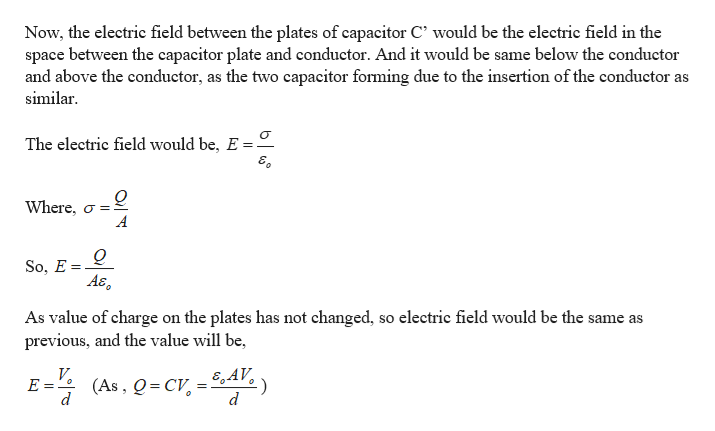# Imagine that two parallel plates are charged to achieve a voltage difference Vo between the plates. They have area A and spacing d. The plates are then electrically isolated so that no charge can be added or taken away. A good conductor of thickness d/2 is placed in between the two plates, as shown. Given that the charge on the plates cannot change, what is the electric field in the regions between the plates, but above and below the conductor? (If you are stuck, Gauss’s Law may help.)

Question
1 views

Imagine that two parallel plates are charged to achieve a voltage difference Vo between the plates. They have area A and spacing d. The plates are then electrically isolated so that no charge can be added or taken away. A good conductor of thickness d/2 is placed in between the two plates, as shown. Given that the charge on the plates cannot change, what is the electric field in the regions between the plates, but above and below the conductor? (If you are stuck, Gauss’s Law may help.)

check_circle

Step 1

Given:

Initial voltage difference between the plate = V0

Distance between the plates = d

Plate area = A

Step 2

Calculating the electric field between the plates:

If a conductor is introduced between the paltes of a capacitor C. The charges will induced on the surface of the conductor. As you can see in the figure, the given capacitor can now be considered as a combination of two capacitors in series.

The charge and plate area for the new capacitors C’ won’t change but the distance between the plate would change.

Here, d’ = d/4 , as the width of the conductor is d/2

Step 3

Calculating the electric field in the spac...help_outlineImage TranscriptioncloseNow, the electric field between the plates of capacitor C' would be the electric field in the space between the capacitor plate and conductor. And it would be same below the conductor and above the conductor, as the two capacitor forming due to the insertion of the conductor as similar The electric field would be, E = Where, o So, E = A& As value of charge on the plates has not changed, so electric field would be the same as previous, and the value will be, V E = E AV (As, Q CV d fullscreen

### Want to see the full answer?

See Solution

#### Want to see this answer and more?

Solutions are written by subject experts who are available 24/7. Questions are typically answered within 1 hour.*

See Solution
*Response times may vary by subject and question.
Tagged in

### Electrostatic Potential and Capacitance